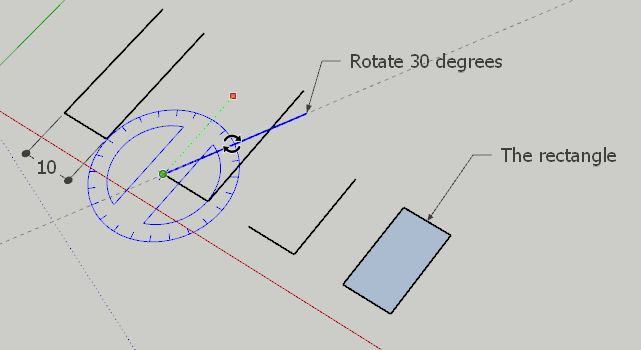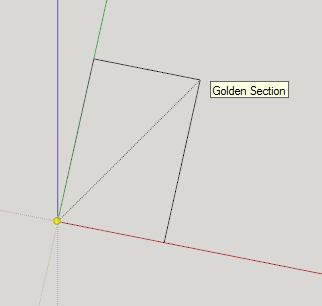# Using angles to set proportions

Hello,

I’m new here and have a very specific question.

I have been reading a book on Pythagorean ratios and their application to rectilinear and curvilinear forms. The key is using angles to determine the ratios of length to side. For example: a rectangle would be defined by its base and the angle from, say, the bottom-left to the top-right corner. The same type of approach is used to draw ellipses.

Concretely, the base might be 10 and the angle 30 degrees. A rectangle results. Or the base might be given as 3 and the angle, 45 degrees, for a square.

Is SketchUp able to do this?

Many thanks,
Stephen

Okay, I discovered the protractor so I’m nearly there…

You can also use the Rotate tool to swing an edge around 30 degrees.-Gully

If you’re not already aware, SketchUp’s inference mechanism allows you to use the rectangle tool to use the Golden Ratio:1 Like

Thanks to you both for the quick responses! Much appreciated. The Rotate tool looks as though it might be the simplest to use in this case.

For info, the ratios are based on the ratios found in Pythagorean tuning (in one key) applied to forms. For example, the ratio 2/3 is a dominant (fifth) the frequency is 1.5 times higher than the tonic. The same thinking is applied to forms using the right angle as the tonic, so the dominant would be 60 degrees, the “octave”, 45 degrees giving a square. The Golden Section is just one case but using angles allows me to combine many of these “harmonics” into one drawing.

I was assuming SketchUp would allow me to do this, it was just a question of, “how”?

Thanks,
Stephen

I realise you are using make, however if able to use pro, you can make a Dynamic Component to create the forms

rect_from_base @ angle.skp (10.3 KB)

you can however use the options for these two uploaded